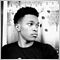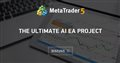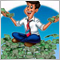# Discussion of article "Neural Networks Made Easy" - page 21423

Nelson Wanyama:
Kept throwing memory leaks. I'm working on my own model rynna

The model is ready. Please find the source and a simple working example from https://www.mql5.com/en/forum/338341/page4#comment_17770620

It is an ongoing project. You might want to visit the thread once in a while to get some amazing upgrades that are still under development.The Ultimate AI EA Project
• 2020.07.14
• www.mql5.com
Hello everyone. I would like to call upon every worthy programmer and trader to a crucial mission...5

Hi Dmitriy,

I have been working on the first two parts of your "Neural Networks" Series for a few weeks now, and I keep comming accros the following code below when cycling through Neurons (or other things).

I really don't get it - in my view this would always leave out the last element. The Total() functions should return a count, then your remove one AND use "<" in the for loop. So for a Total() count of 2, you would only run the for loop once.

What am I missing?

Regards,

Delf

```   int total=Layer.Total()-1;
for(int n=0; n<total; n++)
{
CNeuron *neuron=Layer.At(n);
resultVals.Add(neuron.getOutputVal());
}```66

in some piece of codes you have written something like:

```int total=Layer.Total()-1;
for(int n=0; n<total; n++)
{
CNeuron *neuron=Layer.At(n);
resultVals.Add(neuron.getOutputVal());
}
}```

but assume we have 20 neurons in the layer, and we assign total variable with 19 [int total=Layer.Total()-1] so now total is equal to 19 , when we iterating over neurons from index 0-18 (total=19) we never reach the index 19 (last neuron), I think we must change the code with:

```int total=Layer.Total();
for(int n=0; n<=total; n++)
{
CNeuron *neuron=Layer.At(n);
resultVals.Add(neuron.getOutputVal());
}
}```

or

```int total=Layer.Total()-1;
for(int n=0; n<=total; n++)
{
CNeuron *neuron=Layer.At(n);
resultVals.Add(neuron.getOutputVal());
}
}```

to reach the last neuron on the layer.

what is you idea?27176

Hedayat Yazdani:

in some piece of codes you have written something like:

but assume we have 20 neurons in the layer, and we assign total variable with 19 [int total=Layer.Total()-1] so now total is equal to 19 , when we iterating over neurons from index 0-18 (total=19) we never reach the index 19 (last neuron), I think we must change the code with:

or

to reach the last neuron on the layer.

what is you idea?

At every layer we add one more neuron for bayesian shift. So at calling function to create layer with 20 neurons we creating 21 neurons. And Layer.Total() will return 21. But input data will has only 20 elements. Last bayesian neuron always have '1' in output. So I use Layer.Total()-1. In loop I use "<" because first element of the array has index '0'.